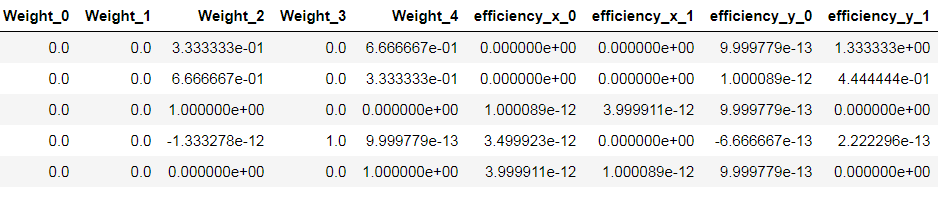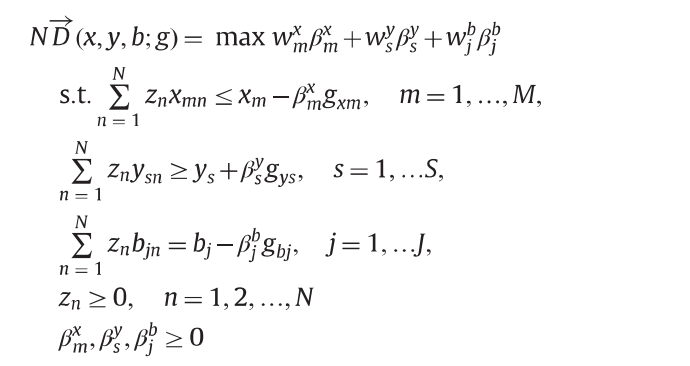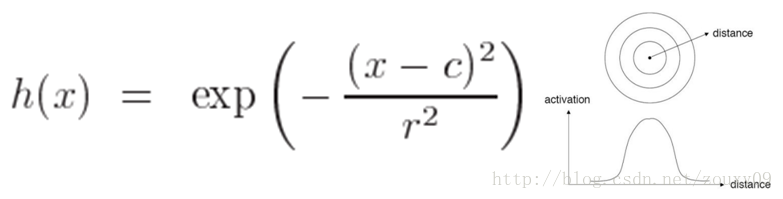• 径向距离函数
千次阅读 多人点赞
2020-07-08 15:05:55

点赞发Nature
关注中Science

上一版非径向距离函数在这
普通非径向距离函数

现在很多研究在测度效率时要考虑非期望产出，如环境技术中CO2排放，银行业中的不良贷款等，这里我写了一个考虑非期望产出的非径向距离函数

import numpy as np
import pandas as pd
import pulp

class DEAProblem:
def __init__(
self,
inputs,
outputs,
weight_vector,
directional_factor=None,
returns="CRS",
disp="weak disposability",
in_weights=[0, None],
out_weights=[0, None],
):
self.inputs = inputs
self.outputs = outputs
self.returns = returns
self.weight_vector = (
weight_vector  # weight vector in directional distance function
)
self.disp = disp

self.J, self.I = self.inputs.shape  # no of DMUs, inputs
_, self.R = self.outputs.shape  # no of outputs
self._i = range(self.I)  # inputs
self._r = range(self.R)  # outputs
self._j = range(self.J)  # DMUs
if directional_factor == None:
self.gx = self.inputs
self.gy = self.outputs
else:
self.gx = directional_factor[: self.I]
self.gy = directional_factor[self.I : (self.I + self.J)]
self.gy = directional_factor[(self.I + self.J) :]

self._in_weights = in_weights  # input weight restrictions
self._out_weights = out_weights  # output weight restrictions

# creates dictionary of pulp.LpProblem objects for the DMUs
self.dmus = self._create_problems()

def _create_problems(self):
"""
Iterate over the DMU and create a dictionary of LP problems, one
for each DMU.
"""

dmu_dict = {}
for j0 in self._j:
dmu_dict[j0] = self._make_problem(j0)
return dmu_dict

def _make_problem(self, j0):
"""
Create a pulp.LpProblem for a DMU.
"""

# Set up pulp
prob = pulp.LpProblem("".join(["DMU_", str(j0)]), pulp.LpMaximize)
self.weights = pulp.LpVariable.dicts(
"Weight", (self._j), lowBound=self._in_weights
)
self.betax = pulp.LpVariable.dicts(
"scalingFactor_x", (self._i), lowBound=0, upBound=1
)

self.betay = pulp.LpVariable.dicts("scalingFactor_y", (self._r), lowBound=0)

self.betab = pulp.LpVariable.dicts(
"scalingFactor_b", (self._s), lowBound=0, upBound=1
)

# Set returns to scale
if self.returns == "VRS":
prob += pulp.lpSum([self.weights[j] for j in self.weights]) == 1

# Set up objective function
prob += pulp.lpSum(
[(self.weight_vector[i] * self.betax[i]) for i in self._i]
+ [(self.weight_vector[self.I + r] * self.betay[r]) for r in self._r]
+ [
(self.weight_vector[self.I + self.R + s] * self.betab[s])
for s in self._s
]
)

# Set up constraints
for i in self._i:
prob += (
pulp.lpSum(
[(self.weights[j0] * self.inputs.values[j0][i]) for j0 in self._j]
)
<= self.inputs.values[j0][i] - self.betax[i] * self.gx.values[j0][i]
)
for r in self._r:
prob += (
pulp.lpSum(
[(self.weights[j0] * self.outputs.values[j0][r]) for j0 in self._j]
)
>= self.outputs.values[j0][r] + self.betay[r] * self.gy.values[j0][r]
)

if self.disp == "weak disposability":
for s in self._s:  # weak disposability
prob += (
pulp.lpSum(
[
for j0 in self._j
]
)
- self.betab[s] * self.gb.values[j0][s]
)

elif self.disp == "strong disposability":
for s in self._s:  # strong disposability
prob += (
pulp.lpSum(
[
for j0 in self._j
]
)
- self.betab[s] * self.gb.values[j0][s]
)

return prob

def solve(self):
"""
Iterate over the dictionary of DMUs' problems, solve them, and collate
the results into a pandas dataframe.
"""

sol_status = {}
sol_weights = {}
sol_efficiency = {}

for ind, problem in list(self.dmus.items()):
problem.solve()
sol_status[ind] = pulp.LpStatus[problem.status]
sol_weights[ind] = {}
for v in problem.variables():
sol_weights[ind][v.name] = v.varValue
sol_efficiency[ind] = pulp.value(problem.objective)
return sol_status, sol_efficiency, sol_weights


简单说，这是一个考虑弱处置性的NDDF模型，input就是决策单元的输入，output是决策单元的输出，bad_outs是决策单元的非期望产出， weight_vector是计算距离函数的权重向量。

你们可以测试一下，有什么问题、有什么思路都可以联系我交流，我还在持续更新中。

希望以后能做成一个比较完整的开源模块给大家用。

举个例子

X = pd.DataFrame(
np.array(
[
[20, 300],
[30, 200],
[40, 100],
[20, 200],
[10, 400],
[11, 222],
[12, 321],
[14, 231],
]
)
)
y = pd.DataFrame(np.array([, , , , , , , ]))
b = pd.DataFrame(np.array([, , , , , , [-2], [-1]]))
weight = [1 / 6, 1 / 6, 1 / 3, 1 / 3]
names = pd.DataFrame(
["Bratislava", "Zilina", "Kosice", "Presov", "Poprad", "ala", "ba", "ca"],
columns=["DMU"],
)

solve = DEAProblem(X, y, b, weight, disp="weak disposability").solve()

status = pd.DataFrame.from_dict(solve, orient="index", columns=["status"])
efficiency = pd.DataFrame.from_dict(solve, orient="index", columns=["efficiency"])
weights = pd.DataFrame.from_dict(solve, orient="index")
results = pd.concat([names, status, efficiency, weights], axis=1)

print(results.round(decimals=4))

DMU   status  efficiency  Weight_0  Weight_1  Weight_2  Weight_3
0  Bratislava  Optimal      0.9182       0.0       0.0    0.0728       0.0
1      Zilina  Optimal      0.5078       0.0       0.0    0.0830       0.0
2      Kosice  Optimal      0.0000       0.0       0.0    1.0000       0.0
3      Presov  Optimal      0.4663       0.0       0.0    0.0830       0.0
4      Poprad  Optimal      0.0000       0.0       0.0    0.0000       0.0
5         ala  Optimal      0.5646       0.0       0.0    0.0000       0.0
6          ba  Optimal      0.4458       0.0       0.0    0.0000       0.0
7          ca  Optimal      0.0000       0.0       0.0    0.0000       0.0


## Cite the work

Yang, F.; Choi, Y.: Lee, H. Life-cycle data envelopment analysis to measure efficiency and cost-effectiveness of environmental regulation in China’s transport sector. Ecological indicators 2021
Choi, Y.; Yang, F.; Lee, H. On the Unbalanced Atmospheric Environmental Performance of Major Cities in China. Sustainability 2020, 12, 5391. https://doi.org/10.3390/su12135391

————————update 2020/12/4——————————
更新了VRS的计算方法
from

        if self.returns == "VRS":
prob += pulp.lpSum([weight for weight in self.weights]) == 1


to

        if self.returns == "VRS":
prob += pulp.lpSum([self.weights[j] for j in self.weights]) == 1


————————

专注于分享利用python科研的技巧，欢迎一起交流、学习、合作。

关于我的博客内容、其他相关的研究问题，有问题可以下方👇评论、或私信我~

更多相关内容
• 最近在想怎么用python实现非径向距离函数 之前用了pyDEA包https://pypi.org/project/pyDEA/，那个包比较简陋，只有普通的CCR BCC模型。 另一方面，MaxDEA因为是打包好的嘛，所以不够灵活。所以想自己做一个NDDF的...

点赞发Nature
关注中Science

最近在想怎么用python实现非径向距离函数

之前用了pyDEA包https://pypi.org/project/pyDEA/，那个包比较简陋，只有普通的CCR BCC模型。

另一方面，MaxDEA因为是打包好的嘛，所以不够灵活。所以想自己做一个NDDF的模型出来。

所以用pyDEA的初始代码进行了一些改造，直接上代码：

import numpy as np
import pandas as pd
import pulp

class DEAProblem:
def __init__(self, inputs, outputs, weight_vector, directional_factor=None, returns='CRS',
in_weights=[0, None], out_weights=[0, None]):
self.inputs = inputs
self.outputs = outputs
self.returns = returns
self.weight_vector = weight_vector # weight vector in directional distance function

self.J, self.I = self.inputs.shape  # no of DMUs, inputs
_, self.R = self.outputs.shape  # no of outputs
self._i = range(self.I)  # inputs
self._r = range(self.R)  # outputs
self._j = range(self.J)  # DMUs
if directional_factor == None:
self.gx = self.inputs
self.gy = self.outputs
else:
self.gx = directional_factor[:self.I]
self.gy = directional_factor[-self.J:]

self._in_weights = in_weights  # input weight restrictions
self._out_weights = out_weights  # output weight restrictions

# creates dictionary of pulp.LpProblem objects for the DMUs
self.dmus = self._create_problems()

def _create_problems(self):
"""
Iterate over the DMU and create a dictionary of LP problems, one
for each DMU.
"""

dmu_dict = {}
for j0 in self._j:
dmu_dict[j0] = self._make_problem(j0)
return dmu_dict

def _make_problem(self, j0):
"""
Create a pulp.LpProblem for a DMU.
"""

# Set up pulp
prob = pulp.LpProblem("".join(["DMU_", str(j0)]), pulp.LpMaximize)
self.weights = pulp.LpVariable.dicts("Weight", (self._j),
lowBound=self._in_weights)
self.betax = pulp.LpVariable.dicts("scalingFactor_x", (self._i),
lowBound=0)

self.betay = pulp.LpVariable.dicts("scalingFacotr_y", (self._r),
lowBound=0)

# Set returns to scale
if self.returns == "VRS":
prob += pulp.lpSum([weight for weight in self.weights]) == 1

# Set up objective function
prob += pulp.lpSum([(self.weight_vector[i]*self.betax[i]) for i in self._i]+[(self.weight_vector[self.I+r]*self.betay[r]) for r in self._r])

# Set up constraints
for i in self._i:
prob += pulp.lpSum([(self.weights[j0]*
self.inputs.values[j0][i]) for j0 in self._j]) <= self.inputs.values[j0][i]-self.betax[i]*self.gx.values[j0][i]
for r in self._r:
prob += pulp.lpSum([(self.weights[j0]*
self.outputs.values[j0][r]) for j0 in self._j]) >= self.outputs.values[j0][r]+self.betay[r]*self.gy.values[j0][r]

return prob

def solve(self):
"""
Iterate over the dictionary of DMUs' problems, solve them, and collate
the results into a pandas dataframe.
"""

sol_status = {}
sol_weights = {}
sol_efficiency = {}

for ind, problem in list(self.dmus.items()):
problem.solve()
sol_status[ind] = pulp.LpStatus[problem.status]
sol_weights[ind] = {}
for v in problem.variables():
sol_weights[ind][v.name] = v.varValue
sol_efficiency[ind] = pulp.value(problem.objective)
return sol_status, sol_efficiency, sol_weights

solve = DEAProblem(X, y, weight).solve()该模块依据了这个公式：文章是张宁老师13年发表在RESR上的综述，

该模块可以自行设定投入X, 产出Y, 方向向量g, 以及权重向量w

后续还得考虑把undesirable output也加入进去。

我在编程的时候发现产出扩张系数是有可能大于1的，我以前一直以为扩张系数就是无效率值，发现还是有些不一样的。

——————update——————
考虑非期望产出的NDDF
考虑强\弱处置性的非期望产出NDDF

## Cite the work

Yang, F.; Choi, Y.: Lee, H. Life-cycle data envelopment analysis to measure efficiency and cost-effectiveness of environmental regulation in China’s transport sector. Ecological indicators 2021
Choi, Y.; Yang, F.; Lee, H. On the Unbalanced Atmospheric Environmental Performance of Major Cities in China. Sustainability 2020, 12, 5391. https://doi.org/10.3390/su12135391

————————

专注于分享利用python科研的技巧，欢迎一起交流、学习、合作。

关于我的博客内容、其他相关的研究问题，有问题可以下方👇评论、或私信我~

展开全文• 任意一个满足Φ（x）=Φ(‖x‖)特性的函数Φ都叫做径向基函数，标准的一般使用欧氏距离（也叫做欧式径向基函数），尽管其他距离函数也是可以的。在神经网络结构中，可以作为全连接层和ReLU层的主要函数。
• ## 径向基函数（RBF）

千次阅读 2020-11-06 09:06:00
理解RBF网络的工作原理可从两种不同的观点出发： （1）当用RBF网络解决非线性映射问题时，用函数逼近与内插的观点来...径向函数技术则是20世纪80年代后期，Powell在解决“多变量有限点严格（精确）插值问题”时引人的

径向基函数是某种沿径向对称的标量函数，通常定义为样本到数据中心之间径向距离（通常是欧氏距离）的单调函数（由于距离是径向同性的）。
理解RBF网络的工作原理可从两种不同的观点出发：
（1）当用RBF网络解决非线性映射问题时，用函数逼近与内插的观点来解释，对于其中存在的不适定(参考中有讲解）问题，可用正则化理论来解决；
（2）当用RBF网络解决复杂的模式分类任务时，用模式可分性观点来理解比较方便，其潜在合理性基于Cover（参考中有讲解）关于模式可分的定理。下面阐述基于函数逼近与内插观点的工作原理。

1963年Davis提出高维空间的多变量插值理论。径向基函数技术则是20世纪80年代后期，Powell在解决“多变量有限点严格（精确）插值问题”时引人的，目前径向基函数已成为数值分析研究中的一个重要领域。
考虑一个由N维输人空间到一维输出空间的映射。设N维空间有P个输人向量，P=1，2，…，P，它们在输出空间相应的目标值为d^p，p=1,2,…,P，P对输入输出样本构成了训练样本集。插值的目的是寻找一个非线性映射函数F(X),使其满足下述插值条件：
（1）函数F描述了一个插值曲面，所谓严格插值或精确插值，是一种完全内插，即该插值曲面必须通过所有训练数据点。
采用径向基函数技术解决插值问题的方法是，选择P个基函数个训练数据，各基函数的形式为：式中，基函数fai为非线性函数，训练数据点xp是fai的中心。基函数以输人空间的点x与中心x^p的距离作为函数的自变量。由于距离是径向同性的，故函数被称为径向基函数。基于径向基函数技术的差值函数定义为基函数的线性组合：在这里需要解释一下||x-xp||这是范数，在平面几何的向量来说就是模，然而一旦维度很高就不知道是什么东西了，可能是衡量距离的一个东西，那么这个代表什么意思呢？简单来说就是一个圆而已，在二维平面，x^p就是圆心，x就是数据了，这个数据距离圆心的距离，因为和数据的位置和大小无关，只和到圆心的半径有关，况且同一半径圆上的点到圆心是相等的因此取名为径向，代入映射函数就是径向基函数了，我们看看径向基函数有什么特点：
将（1）式的插值条件代入上式，得到P个关于未知系数w^p，p = 1,2,…,P的线性方程组：则上述方程组可改写为：（其中第一个矩阵下标存在错误，相信你能看懂）令大fai表示元素fai ip的PxP阶矩阵，w和d分别表示系数向量和期望输出向量，（5）式还可以写成下面的向量形式：式中，大fai称为插值矩阵，若大fai为可逆矩阵，就可以从（6）式中解出系数向量w，即：通过上面大家可以看到为了使所有数据都在曲面还需要系数调节，此时求出系数向量就求出了整个的映射函数了。

RBF 和 BP network 区别：
BP神经网络的隐节点采用输入模式与权向量的内积作为激活函数的自变量，而激活函数采用Sigmoid函数。各隐节点对BP网络的输出具有同等地位的影响，因此BP神经网络是对非线性映射的全局逼近。

RBF神经网络的隐节点采用输入模式与中心向量的距离（如欧式距离）作为函数的自变量，并使用径向基函数（如Gaussian函数）作为激活函数。神经元的输入离径向基函数中心越远，神经元的激活程度就越低。RBF网络的输出与数据中心离输入模式较劲的“局部”隐节点关系较大，RBF神经网络因此具有“局部映射”特性。

RBF网络：神经元是一个以gaussian函数（或者其他）为核函数的神经元。
RBF Network 通常只有三层。 输入层、中间层计算输入 x 矢量与样本矢量 c 欧式距离的 Radial Basis Function (RBF) 的值，输出层计算它们的线性组合。第一阶段为非监督学习，从数据中选取记忆样本。例如聚类算法可在该阶段使用。
第二阶段为监督学习，训练记忆样本与样本输出的联系。该阶段根据需要可使用BP。

简单说明一下为什么RBF网络学习收敛得比较快。当网络的一个或多个可调参数（权值或阈值）对任何一个输出都有影响时，这样的网络称为全局逼近网络。由于对于每次输入，网络上的每一个权值都要调整，从而导致全局逼近网络的学习速度很慢。BP网络就是一个典型的例子。
如果对于输入空间的某个局部区域只有少数几个连接权值影响输出，则该网络称为局部逼近网络。常见的局部逼近网络为RBF网络。

隐藏层的作用是把向量从低维映射到高维，低维线性不可分的情况到高维就线性可分了戳这里理解）。

参考

展开全文神经网络
• ## 径向基函数

千次阅读 2019-11-15 09:34:36
径向函数是一个取值仅仅依赖于离原点距离的实值函数，也就是Φ（x）=Φ(‖x‖),或者还可以是到任意一点c的距离，c点称为中心点，也就是Φ（x，c）=Φ(‖x-c‖)。任意一个满足Φ（x）=Φ(‖x‖)特性的函数Φ都叫做...

百度百科：
径向基函数是一个取值仅仅依赖于离原点距离的实值函数，也就是Φ（x）=Φ(‖x‖),或者还可以是到任意一点c的距离，c点称为中心点，也就是Φ（x，c）=Φ(‖x-c‖)。任意一个满足Φ（x）=Φ(‖x‖)特性的函数Φ都叫做径向基函数，标准的一般使用欧氏距离（也叫做欧式径向基函数）。

https://blog.csdn.net/zouxy09/article/details/13297881
https://www.cnblogs.com/zhangchaoyang/articles/2591663.html展开全文• 径向距离函数 考虑非期望产出的非径向距离函数 这一版更新比较简单，增加了强处置性假设下的非径向距离函数（NDDF）计算。比较强\弱处置假设下的NDDF，可以计算相应的环境规制成本[ Technological Forecasting &...
• RBF径向基函数 (RBF) 插值使用标准或自定义距离函数为任意维度的输入和输出值构建径向基函数。安装\$ npm install rbf用法 var RBF = require ( 'rbf' ) ;var points = [ [ 0 , 0 ] , [ 0 , 100 ]] ;// values could ...JavaScript
• DDF方向距离函数--文献文献介绍内容介绍方向距离函数DDF定义效率评估Directional calculation method(DVS)三级目录 文献介绍 文献名字是：Expected efficiency based on directional distance function in data ...python 线性规划
• ## 径向基函数神经网络（RBFNN）详解

万次阅读 多人点赞 2019-09-13 16:29:05
径向函数神经网络（RBFNN） 前言 RBFNN是20世纪80年代末提出的一种单隐层、以函数逼近为基础的前馈神经网络。随着研究日渐成熟，RBFNN以其结构简单、非线性逼近能力强以及良好的推广能力，受到各领域研究者的极...深度学习 RBFNN 神经网络
• 以上是对核函数的介绍，在本篇文章中将重点介绍径向函数，这是一个非常简单但常见的核。 线性回归和 RBF（径向函数） 在回归问题中，我们试图估计从 X 推断 Y 的最佳函数。如果 X 和 Y 之间存在非线性关系，则...机器学习 深度学习 核方法 svm
• ## 径向分布函数

万次阅读 热门讨论 2018-10-14 17:22:15C++ 算法
• 径向函数RBF2006-12-12 北京科技大学 付冬梅 * 例 建立一个径向基神经网络，对非线性函数y=sqrt(x)进行逼近，并作出网络的逼近误差曲线。 6-7 RBF网络的MATLAB函数及功能 2006-12-12 北京科技大学 付冬梅 * 误差...
• ## RBF径向基函数

万次阅读 多人点赞 2018-07-02 19:10:19
一、径向基函数径向基函数是某种沿径向对称的标量函数，通常定义为样本到数据中心之间径向距离（通常是欧氏距离）的单调函数（由于距离是径向同性的）。RBF核是一种常用的核函数。它是支持向量机分类中最为常用的核...
• 将RBF用于插值标签(空格分隔)：径向函数插值 算法 RBF曲面重构当高维数据稀疏，需要预测一些数据，需要使用曲面重构的方法。 曲面重构一般可以分为：插值重构曲面插值里我们一般使用径向函数插值。RBF (Radial ...
• 径向函数神经网络是不同于BP神经网络的另一种的前馈神经网络，由输入层、一层非线性隐层（径向基层）和线性输出层组成的。 关于径向函数神经网络，首先要介绍一个定理，cover定理，对于一个复杂的在低维空间...
• ## 高斯径向基函数(RBF)神经网络

万次阅读 多人点赞 2019-04-03 00:53:52
高斯径向函数(RBF)神经网络 牛顿插值法-知乎 ...说径向基网络之前，先聊下径向函数径向函数（英语：radial basis function，缩写为RBF）是一个取值仅依赖于到原点距离的实值函数，即 ϕ(x...RBF 机器学习
• 这张图片的实际含义是以坐标原点为参考点，距离原点距离x处的小球个数f(x)分布图。这个问题理解起来好容易，统计距离和小球个数的关系，but实际问题是过冷水要处理的是这样的问题请问请告诉我随意找一个小球为原点，...
• ## 基于径向基函数(RBF)的函数插值

千次阅读 多人点赞 2020-07-07 01:16:19
1. 函数插值 2. RBF函数插值 代码实现
• [lammps第十四讲]lammps输出RDF（径向分布函数）详解及示例教程 原创一直陪着你的LAMMPS交流站2021-10-18 11:40 收录于话题#lammps案例16个内容 大家好，小编最近的课题需要输出RDF（径向分布函数），小编就去...
• 以上是对核函数的介绍，在本篇文章中将重点介绍径向函数，这是一个非常简单但常见的核。 线性回归和 RBF（径向函数） 在回归问题中，我们试图估计从 X 推断 Y 的最佳函数。如果 X 和 Y 之间存在非线性关系，则...算法 python 机器学习 人工智能 深度学习
• 之前写过径向函数(RBF)神经网络做分类或者拟合。然后挖了个坑说在《Phase-Functioned Neural Networks for Character Control》里面提到了用于做地形编辑，所以这篇博客就是解析一下如何用RBF做网格编辑系统。 ...
• Malmquist-Lenberger指数（ML指数）是距离函数与DEA计算中常用的指数，ML指数可以进一步分解为技术进步technological progress和效率改善effeciency change. 技术进步常被当做增长模型中的全要素生产率，用到许多...python
• 这几天，用PYTHON写了一个计算SiO2的O-Si间的偏径向分布函数(partial RDF)和针对不同Si近邻(neighbor1，neighbor2...neighbor6)的偏偏径向分布函数(partial partial RDF)，自己起的名字。如图1，以此可以枚举SiO2...知识图谱 机器学习 人工智能...